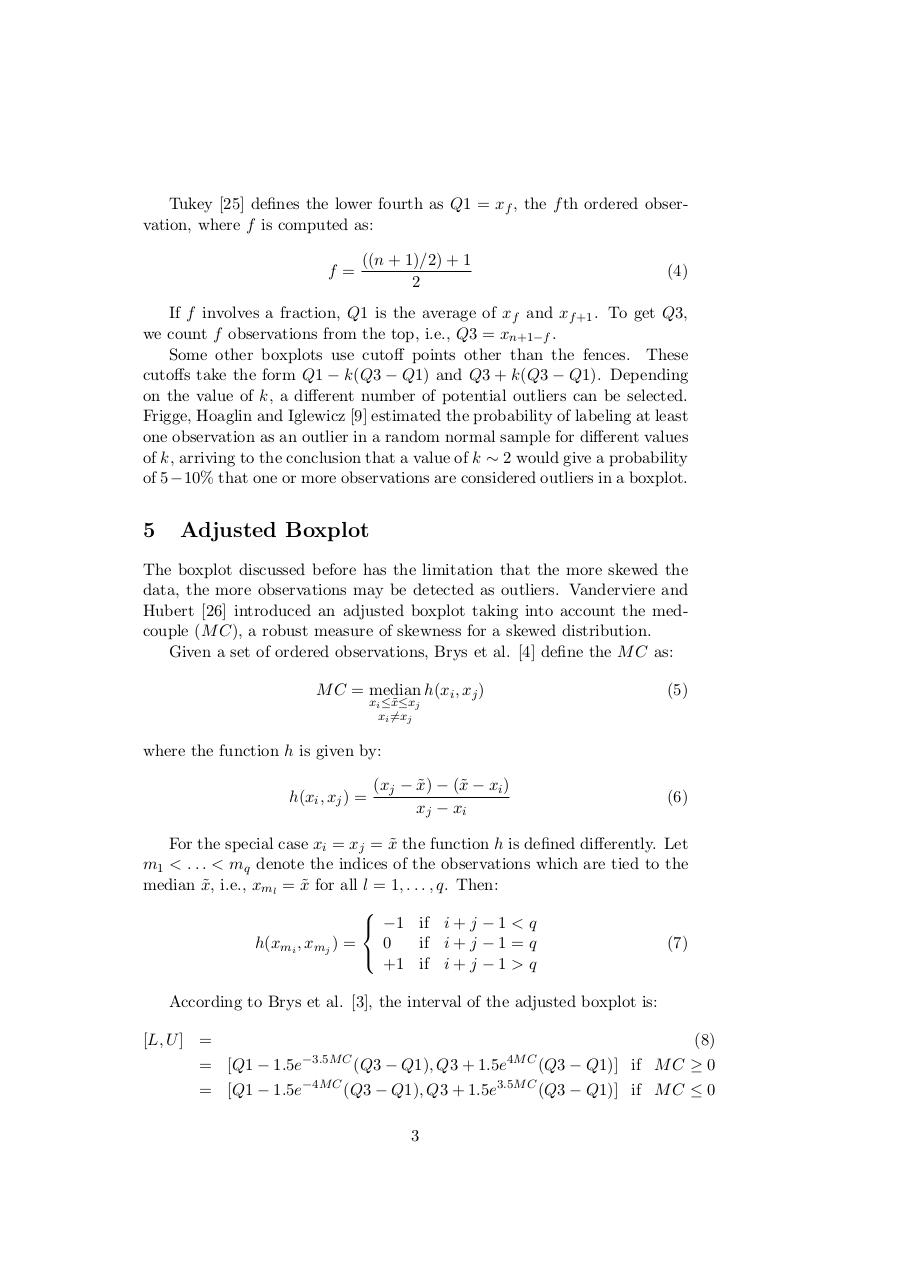# PDF Archive

Easily share your PDF documents with your contacts, on the Web and Social Networks.

## Outlier Methods external.pdfPage 1 2 34516

#### Text preview

Tukey  defines the lower fourth as Q1 = xf , the f th ordered observation, where f is computed as:
f=

((n + 1)/2) + 1
2

(4)

If f involves a fraction, Q1 is the average of xf and xf +1 . To get Q3,
we count f observations from the top, i.e., Q3 = xn+1−f .
Some other boxplots use cutoff points other than the fences. These
cutoffs take the form Q1 − k(Q3 − Q1) and Q3 + k(Q3 − Q1). Depending
on the value of k, a different number of potential outliers can be selected.
Frigge, Hoaglin and Iglewicz  estimated the probability of labeling at least
one observation as an outlier in a random normal sample for different values
of k, arriving to the conclusion that a value of k ∼ 2 would give a probability
of 5−10% that one or more observations are considered outliers in a boxplot.

5

The boxplot discussed before has the limitation that the more skewed the
data, the more observations may be detected as outliers. Vanderviere and
Hubert  introduced an adjusted boxplot taking into account the medcouple (M C), a robust measure of skewness for a skewed distribution.
Given a set of ordered observations, Brys et al.  define the M C as:
M C = median h(xi , xj )

(5)

xi ≤˜
x≤xj
xi 6=xj

where the function h is given by:
h(xi , xj ) =

(xj − x
˜) − (˜
x − xi )
xj − xi

(6)

For the special case xi = xj = x
˜ the function h is defined differently. Let
m1 &lt; . . . &lt; mq denote the indices of the observations which are tied to the
median x
˜, i.e., xml = x
˜ for all l = 1, . . . , q. Then:

 −1 if i + j − 1 &lt; q
0
if i + j − 1 = q
h(xmi , xmj ) =
(7)

+1 if i + j − 1 &gt; q
According to Brys et al. , the interval of the adjusted boxplot is:
[L, U ] =

(8)
−3.5M C

= [Q1 − 1.5e

−4M C

= [Q1 − 1.5e

4M C

(Q3 − Q1)] if M C ≥ 0

3.5M C

(Q3 − Q1)] if M C ≤ 0

(Q3 − Q1), Q3 + 1.5e

(Q3 − Q1), Q3 + 1.5e
3